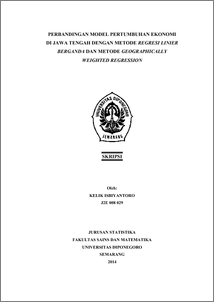# PERBANDINGAN MODEL PERTUMBUHAN EKONOMI DI JAWA TENGAH DENGAN METODE REGRESI LINIER BERGANDA DAN METODE GEOGRAPHICALLY WEIGHTED REGRESSION

ISBIYANTORO, KELIK (2014) PERBANDINGAN MODEL PERTUMBUHAN EKONOMI DI JAWA TENGAH DENGAN METODE REGRESI LINIER BERGANDA DAN METODE GEOGRAPHICALLY WEIGHTED REGRESSION. Undergraduate thesis, FSM Undip.Preview
PDF
744Kb

## Abstract

One of the equipments to see the success of the Government in economics field is the economic growth. To see the economic growth of a region, can be seen from the growth of region Gross Domestic Product (GDP). All this time, the economic growth is often modeled by multiple linear regression, whereas the model describes the general conditions. In fact, there are differences such as geographical factor, socio-cultural circumstance, and the other matters. This allows the appearance of spatial heterogenity in the regression parameters, to overcomes it, the OLS (Ordinary Least Square) regression is developed into Georaphically Weighted Regression (GWR). This model is a local linear regression model that generates local estimator model parameters for each point or location where the data is collected. This research discusses the factors that effect the economic growth in Central Java. The model suitability testing result shows that there is no differences in multiple linear regression model and GWR model toward the economic growth in Central Java. Results of the research shows there are three variables that have effect, they are: Total Labor Force, Major MSEs, and the number of markets. The three variables have the same effect in each county / city. Keywords: Economic Growth, GDP, Multiple Linear Regression, Geographically Weighted Regression

Item Type: Thesis (Undergraduate) H Social Sciences > HA Statistics Faculty of Science and Mathematics > Department of Statistics 43675 Mr Hasbi Yasin 10 Sep 2014 15:40 10 Sep 2014 15:40

Repository Staff Only: item control page